The displacement of a particle as a function of time is shown in the figure. The figure shows that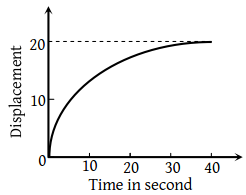1. The particle starts with certain velocity but the motion is retarded and finally the particle stops

2. The velocity of the particle is constant throughout

3. The acceleration of the particle is constant throughout.

4. The particle starts with constant velocity, then motion is accelerated and finally the particle moves with another constant velocity

Concept Questions :-

Graphs
High Yielding Test Series + Question Bank - NEET 2020

Difficulty Level:

A ball is thrown vertically upwards. Which of the following graph/graphs represent velocity-time graph of the ball during its flight (air resistance is neglected)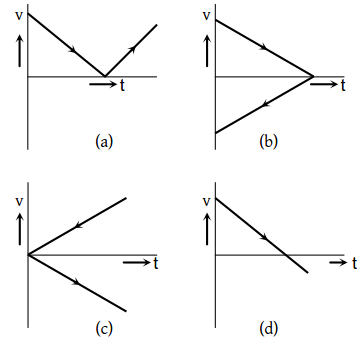1. a

2. b

3. c

4. d

Concept Questions :-

Uniformly accelerated motion
High Yielding Test Series + Question Bank - NEET 2020

Difficulty Level:

The graph between the displacement x and time t for a particle moving in a straight line is shown in figure. During the interval $OA,\text{\hspace{0.17em}}AB,\text{\hspace{0.17em}}BC$ and CD, the acceleration of the particle is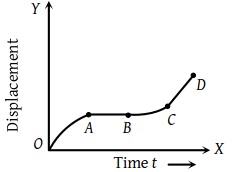OA AB BC CD (1) + 0 + + (2) – 0 + 0 (3) + 0 – + (4) – 0 – 0

Concept Questions :-

Graphs
High Yielding Test Series + Question Bank - NEET 2020

Difficulty Level:

The vt graph of a moving object is given in figure. The maximum acceleration is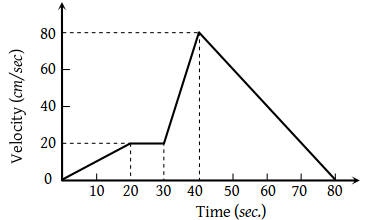1. $1cm/se{c}^{2}$

2. $2cm/se{c}^{2}$

3. $3\text{\hspace{0.17em}\hspace{0.17em}}cm/se{c}^{2}$

4. $6\text{\hspace{0.17em}\hspace{0.17em}}cm/se{c}^{2}$

Concept Questions :-

Graphs
High Yielding Test Series + Question Bank - NEET 2020

Difficulty Level:

The displacement versus time graph for a body moving in a straight line is shown in figure. Which of the following regions represents the motion when no force is acting on the body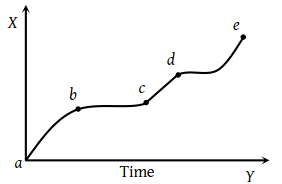(1) ab

(2) bc

(3) cd

(4) de

Concept Questions :-

Graphs
High Yielding Test Series + Question Bank - NEET 2020

Difficulty Level:

The $x-t$ graph shown in figure represents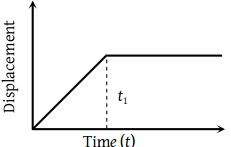(1) Constant velocity

(2) Velocity of the body is continuously changing

(3) Instantaneous velocity

(4) The body travels with constant speed upto time t1 and then stops

Concept Questions :-

Graphs
High Yielding Test Series + Question Bank - NEET 2020

Difficulty Level:

A lift is going up. The variation in the speed of the lift is as given in the graph. What is the height to which the lift takes the passengers ?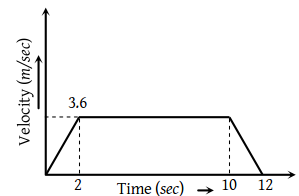(1) 3.6 m

(2) 28.8 m

(3) 36.0 m

(4) Cannot be calculated from the above graph

Concept Questions :-

Graphs
High Yielding Test Series + Question Bank - NEET 2020

Difficulty Level:

The velocity-time graph of a body moving in a straight line is shown in the figure. The displacement and distance travelled by the body in 6 sec are respectively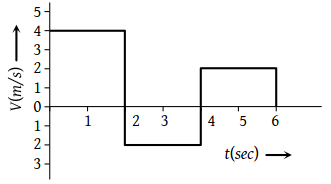(1) 8 m, 16 m

(2) 16 m, 8 m

(3) 16 m, 16 m

(4) 8 m, 8 m

Concept Questions :-

Graphs
High Yielding Test Series + Question Bank - NEET 2020

Difficulty Level:

Velocity-time (v-t) graph for a moving object is shown in the figure. Total displacement of the object during the time interval when there is non-zero acceleration and retardation is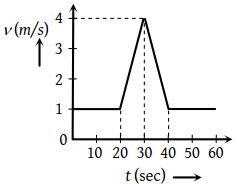(1) 60 m

(2) 50 m

(3) 30 m

(4) 40 m

Concept Questions :-

Graphs
High Yielding Test Series + Question Bank - NEET 2020

Difficulty Level:

For the velocity-time graph shown in figure below the distance covered by the body in last two seconds of its motion is what fraction of the total distance covered by it in all the seven seconds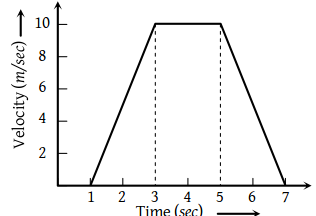(1) $\frac{1}{2}$

(2) $\frac{1}{4}$

(3) $\frac{1}{3}$

(4) $\frac{2}{3}$

Concept Questions :-

Graphs